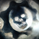Dilihat 9050 kali
9050
Version 2 - Linear Regression Slope. This version will have more freedom on picking your own length for all the Inputs.

One of the main reason I changed it is because, Slope calculation on transition period was not being computed properly. Because the Version 1, looks back the length assigned, and compute the slope based on two candle readings, could be 10 days apart or 50. That was misleading.

Therefore, I changed it to plot daily slope and Smooth it with an EMA .

Linear Regression Curve -
List of All my Indicators - https://www.tradingview.com/p/stocks/?so...
```// Created by UCSgears -- Version 2
// Simple linear regression slope - Good way see if the trend is accelarating or decelarating

study(title="UCSGEARS - Linear Regression Slope", shorttitle="UCS-LRS", overlay=false)

src = close
//Input
clen = input (defval = 50, minval = 1, title = "Curve Length")
slen = input(defval=5, minval=1, title="Slope Length")
glen = input(defval=13, minval=1, title="Signal Length")

//Linear Regression Curve
lrc = linreg(src, clen, 0)
//Linear Regression Slope
lrs = (lrc-lrc)/1
//Smooth Linear Regression Slope
slrs = ema(lrs, slen)
//Signal Linear Regression Slope
alrs = sma(slrs, glen)
//loalrs = sma(slrs, (glen*5))

uacce = lrs > alrs and lrs > 0
dacce = lrs < alrs and lrs < 0

scolor = uacce ? green : dacce ? red : blue

plot(0, title = "Zero Line", color = gray)
plot(slrs, color = scolor, title = "Linear Regression Slope", style = histogram, linewidth = 4)
plot(alrs, color = gray, title = "Average Slope")```gmax
In a nutshell, its the slope of the slope. Take the condition for the color and work with it to add the strategy. If you need help, I can do it over the weekend.
Balasmoveon
Thanks.
The Major glitch on version 1 was Slope was calculated from today and 5 days earlier (with default Setting), During transition period, the dips on curve was ignored, because of the look back was 5 days ago. This should be better.
Balasmoveon
It Depends what you are looking for (short term / Long Term) -

It also depends on the price on the price action. (Steady vs Parabolic)

If you use 20, the LRC (linear regression curve) is more sensitive to tick reading. Creating quicker reaction on the SLOPE.

Remember the equation for linear slope is (y=mx+b) y = y axis, x = x axis, b is just a constant, m is the slope.

Where as for parabolic movers the equation changes by applying integration to this equation to smooth out the Parabolic mover. Its too much of math for those handful of parabolic movers out there. Simple solution would be expand the time frame (reduce it to lower timeframe) and look out for loss of momentum there.

Two highlighted region - The Parabolic Shoot up is not smooth, while the Drop was more linear.
Balasmoveon
To answer Slope Length - Just an EMA to smooth the Daily chops out. Signal is the EMA of the smooth Slope. Its pretty much like how the Stochastics are coded. %K, %D and Signal.
Balas